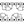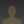0%
0%

# Nextion 3.5" LCD 8 Function Calculator

A useful calculator, similar in style to the Windows 10 standard calculator for the Arduino Uno.

Similar projects worth following
110 views
A useful calculator for the Arduino Uno. The calculator is similar in style to the standard calculator that ships with Windows 10. Note: It does not include the scientific and programmer functions that the Windows 10 calculator does, but these functions may be implemented at a later date. I decided to make it to teach myself how to write a small, top down recursive descent parser and expression evaluator for the Arduino Uno.

The calculator provides a set of 8 mathematical functions:

Percentage calculation

1/x calculation

Square root

Square

[C]ancel - clears the calculator's memory

[CE] Clear Entry - Deletes the last entry made into the calculator

All calculations are carried out with double precision. Note that because of the Arduino being as small as it is, the output of decimals is limited to two places.

HOW THE CODE WORKS

You may have noticed that this calculator isn't like many of the others you have seen. It allows you to enter expressions like `1+3*4` and then executes the expression when you press the equals key. The calculator also follows the mathematical rules for expressions containing multiplication and division operators. The answer to `1+3*4` is `13`, not `16`. Multiplication and division of terms is done before addition and subtraction.

So, how is this magic achieved? It is done using a technique called Top-down, recursive descent parsing. That's quite a mouthful, isn't it? It is also rather tedious to explain and this article would be far too long if I attempted to do so.

If you're interested in learning the technique, there is a series of articles written by Jack Crenshaw called Let's Build A Compiler. One of the articles in this series covers the development of a top-down, recursive descent expression parser, which is what you are after if you are thinking of building your own calculator. The article is very readable and you don't need a degree in Computer Science to understand it like you do with so many other articles on the subject on the Internet. It is located here: https://compilers.iecc.com/crenshaw/tutor2.txt

Don't worry if it takes you more than a few attempts to understand what Jack is talking about. Parsing is hard and requires study. And bare this in mind: it took them years in the 50s to work out how to do this stuff. "So, how long did it take you?" Not telling! :)

BTW if you build a calculator, drop me a message below and let me know where it is. I enjoy looking at them. Good luck!

### calculator-ui.HMI

Nextion Editor HMI file.

hmi - 1.40 MB - 10/07/2020 at 11:47

### calculator-ui.tft

Nextion LCD TFT file. To be uploaded to the Nextion.

tft - 540.74 kB - 10/07/2020 at 11:46

### Nextion-Calculator.ino

Calculator source code for the Arduino

ino - 13.33 kB - 10/07/2020 at 11:45

• 1 × Arduino Uno
• 1 × Itead Nextion NX4832T035 - 3.5" TFT HMI LCD Touch screen LCD display

Share

## Similar Projects

Project Owner Contributor

### EEPROM writer for AT28C256 using Arduino MegaAndre Baptista

Project Owner Contributor

### Using a STM32 like an Arduino Tutorial | STM32F103akarshagarwal98

# Does this project spark your interest?

Become a member to follow this project and never miss any updates